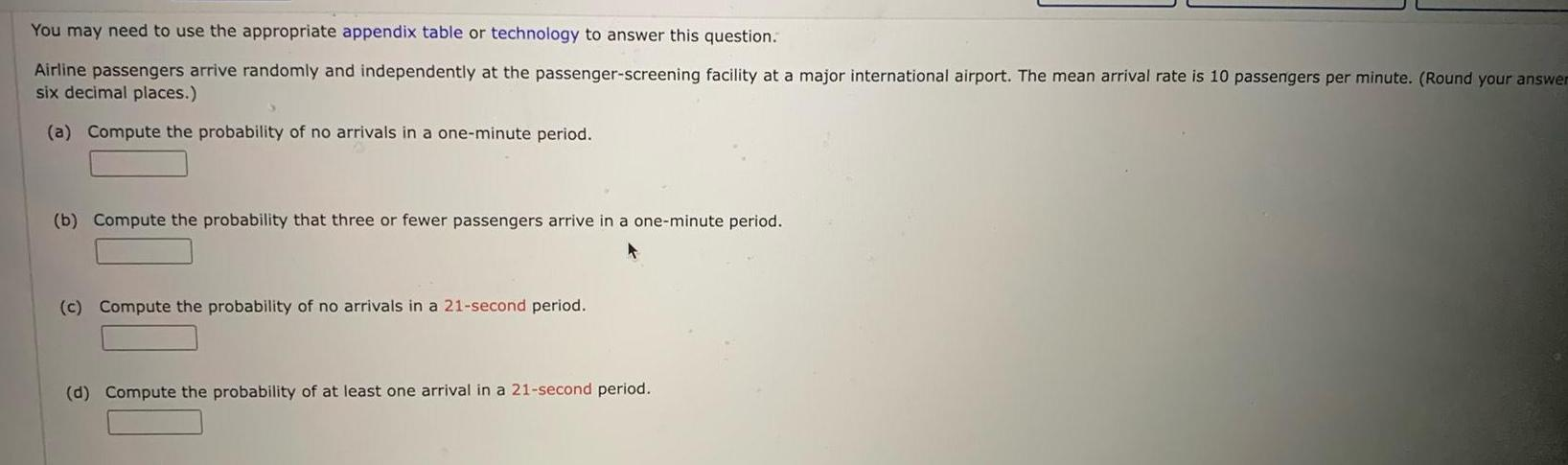Question:

# You may need to use the appropriate appendix table or

Last updated: 11/19/2023You may need to use the appropriate appendix table or technology to answer this question Airline passengers arrive randomly and independently at the passenger screening facility at a major international airport The mean arrival rate is 10 passengers per minute Round your answer six decimal places a Compute the probability of no arrivals in a one minute period b Compute the probability that three or fewer passengers arrive in a one minute period c Compute the probability of no arrivals in a 21 second period d Compute the probability of at least one arrival in a 21 second period